# EM.Ferma Tutorial Lesson 4: Computing The Magnetic Field Of Linear Currents In Free Space & Magnetic Media

Jump to: navigation, search
 Tutorial Project: Computing The Magnetic Field Of Linear Currents In Free Space & Magnetic Media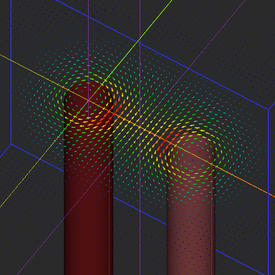Objective: In this project, you will build current-carrying cylindrical objects and compute their magnetic fields in the free space and magnetic material parts. Concepts/Features: Volume Current Source Current Density Vector Magnetic Material Permeability Magnetic Field Magnetic Vector Potential Magnetostatic Analysis Minimum Version Required: All versions

## What You Will Learn

This tutorial will guide you through all the necessary steps required to set up and perform a basic magnetostatic simulation and visualize and graph the simulation results. Specifically, you will create cylindrical current objects and analyze their magnetic field and potential. You will also introduce magnetic material objects with simple and complex geometrical shapes and see the effect of their presence on the magnetic fields.

## Getting Started

Open the EM.Cube application and switch to EM.Ferma. Start a new project with the following parameters:

 Name EMFerma_Lesson4 Millimeters N/A (keep default) N/A (keep default) N/A (keep default)

## Constructing the Geometry of a Current-Carrying Cylinder

Create a volume current source group called "VCS_1" in the navigation tree. Right-click on the Volume Currents item in the navigation tree and select Insert New Current Source... from the contextual menu. In the New current source dialog, set the values of the components of the current density vector as follows:

Component Definition
Jx 0
Jy 0
Jz 1e+6

With the VCS_1 group active, click the Cylinder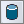button of the Object Toolbar or select the menu item Object → Solid → Cylinder and draw an upright cylinder according to the table below: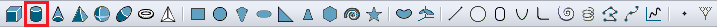Selecting the Cylinder Tool from the Object Toolbar.
Part Object Type Material Type Dimensions Coordinates Rotation Angles
Cylinder_1 Cylinder VCS_1 radius = 2mm, height = 50mm (0, 0, -25mm) (0°, 0°, 0°)

Open the mesh settings dialog and change the fixed mesh cell size to Δx = Δy = Δz = 0.5mm. Define three field sensor observables according to the table below:

Field Sensor Direction Coordinates Plot Type Max. Size Cone Length Ratio Cone Radius Ratio
Sensor_1 Z (0, 0, 0) Vector 0.5 0.75 0.25
Sensor_2 X (0, 0, 0) Vector 2 0.25 0.25
Sensor_3 Y (0, 0, 0) Intensity - - -

Your physical structure should now look like this: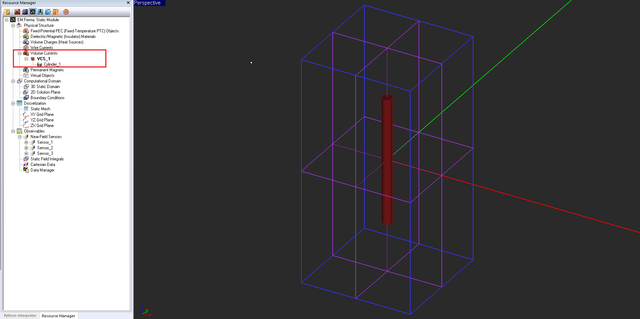The cylindrical current object along with the enclosing domain box and three field sensor planes.

The figure below shows the mesh of the cylindrical object: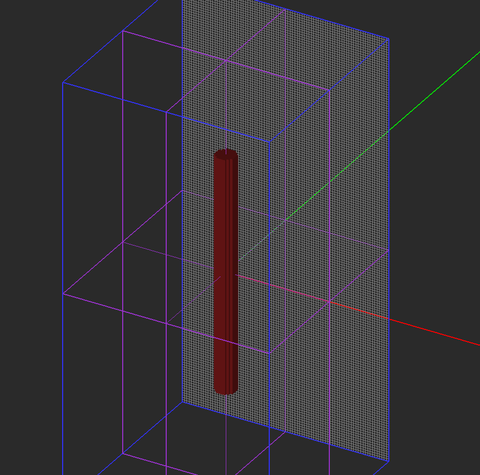The fixed-cell mesh of the cylinder with the ZX mesh grid plane visible behind it.

## Performing a Magnetostatic Analysis of Your Structure

At this time, your project is ready for magnetostatic simulation. You don't have to choose the simulation engine type of electrostatic vs. magnetostatic. If the program detects current sources, it will perform a magnetostatic analysis.EM.Ferma's simulation engine solves a linear system whose solution is the potential values at all the N grid points. An electrostatic linear system involves N unknowns corresponding to the electric scalar potential, while a magnetostatic linear system involves 3N unknowns corresponding to the magnetic vector potential.

At the end of the magnetostatic simulation, visualize the field sensor plots. Note that the electric field components and electric potential are all zero. The top vector plot shown below clearly illustrates the rotational nature of the magnetic field of a linear current and verifies the famous right-hand rule.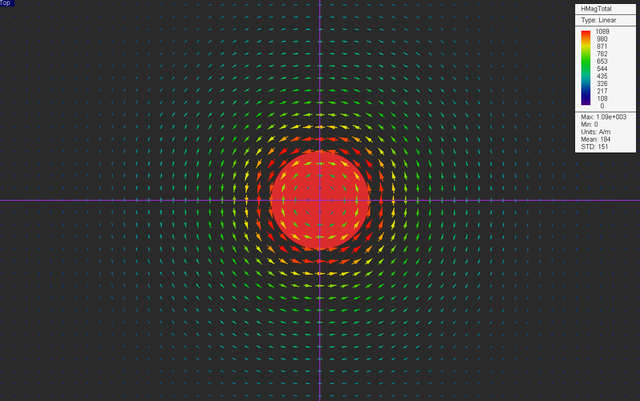The vector plot of the magnetic field distribution in the horizontal Sensor_1 plane.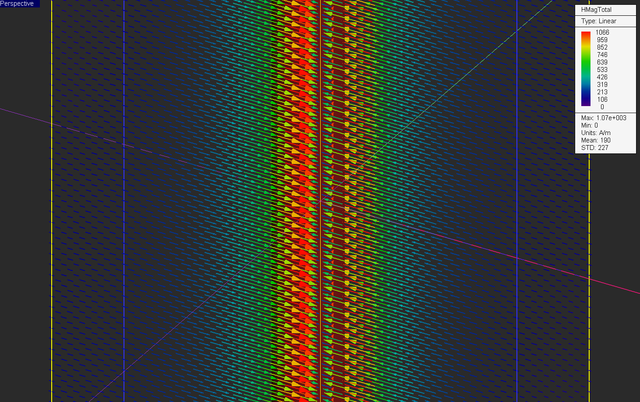The vector plot of the magnetic field distribution in the vertical Sensor_2 plane.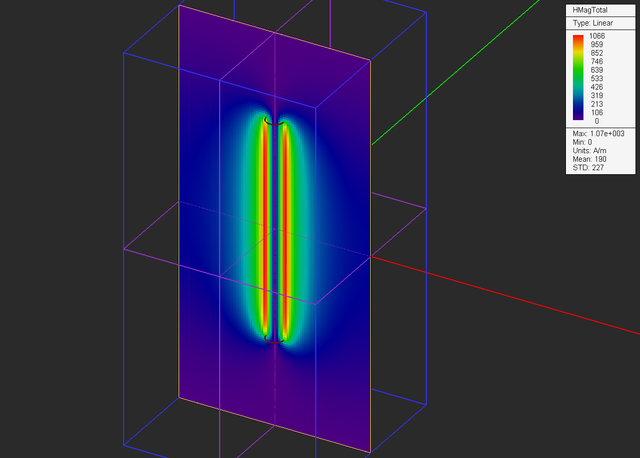The intensity plot of the total magnetic field distribution in the vertical Sensor_3 plane.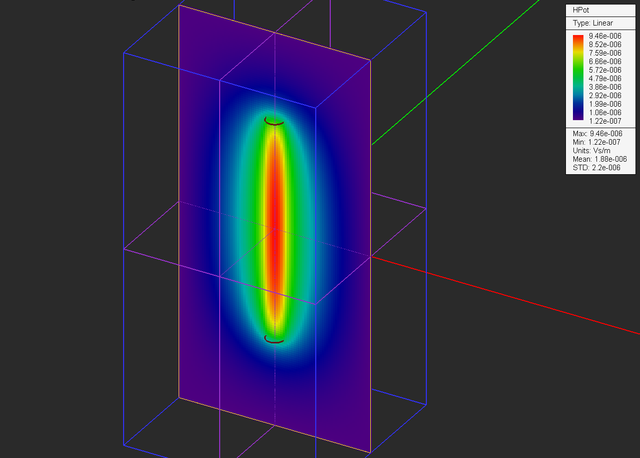The intensity plot of the magnitude of magnetic vector potential distribution in the vertical Sensor_3 plane.

## Verifying Your Magnetostatic Simulation Results

Consider an infinitely long vertical cylindrical current of radius a and a uniform current density $\mathbf{J(r)} = J_0 \mathbf{\hat{z}}$. Due to the symmetry of the cylindrical current, you would expect the magnetic field to be circumferential (azimuthal) and uniform at all spherical observation angles. In other words,

$\mathbf{H(r)} = H_{\phi}(r) \bf{\hat{\phi}}$

Applying Ampere's law on a circular loop of radius r around the current column, one can write:

$\oint_{C} \mathbf{H(r)} . \mathbf{dl} = \int\int_{S} \mathbf{J(r)} . \mathbf{ds}= I_{tot}$

or

$H_{\phi}(r) \left( 2\pi r \right) = J_0 \left( \pi \rho^2 \right)$

where ρ = r if r ≤ a and ρ = a if r > a. Then, one can derive the following relationships for the magnetic field of an infinitely long uniform cylindrical current distribution:

$H_{\phi}(r) = \frac{1}{2}J_0 r, \ \ \ \ \ \ \ r\le a$

$H_{\phi}(r) = \frac{I_0}{2\pi r}, \ \ \ \ \ r\gt a$

where I0 = Itot = πr2J0 is the total current flowing through the cylinder. For your cylindrical object, I0 = π(0.002)2(102) = 12.566A. At two distance of r = 2mm and r = 10mm from the cylinder's axis, you will find:

$H_{\phi}(r = 0.002m) = (0.5)(10^6)(0.002m) = 1000A/m$

$H_{\phi}(r = 0.01m) = \frac{12.566A}{(2\pi)(0.01m)} = 200A/m$

Next, open the Data manager and plot the data file “Sensor_1_X_HTotal.DAT”. As you can see from the figure below, for 0 < r ≤ a = 2mm, the field varies linearly with the distance, while for r > a, it drops as 1/r.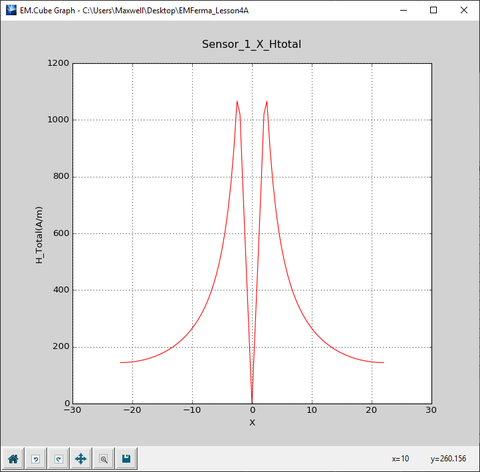The 2D Cartesian graph of variation of the magnetic along the X direction on the center horizontal plane.

Open the data file “Sensor_1_X_HTotal.DAT” in the data manager's spreadsheet and view its contents. The computed total magnetic field values at r = 2mm and r = 10mm are 1020A/m and 264A/m, respectively. The simulated field value at r = 10mm is slightly different than the theoretical value because the above analytical derivations were based on the assumption of an infinitely long cylinder. Your cylinder object in this project has a finite length, and the finite edge effects become more pronounced as you move farther away from the cylinder's axis.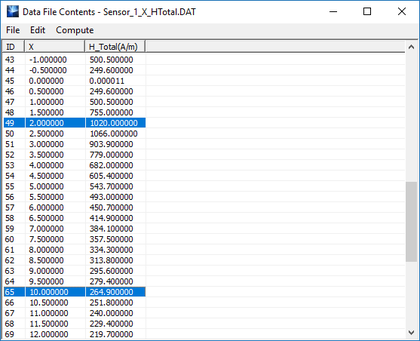The contents of the data file “Sensor_1_X_HTotal.DAT” shown in the data manager's spreadsheet.

## Analyzing Two Parallel Line Currents

In this part of the tutorial lesson, you will add another current-carrying cylinder to your structure but with its current flowing in the opposite direction. Define a new volume current source group called "VCS_2" with a lighter brown color. Set the values of the components of the current density vector as follows:

Component Definition
Jx 0
Jy 0
Jz -1e+6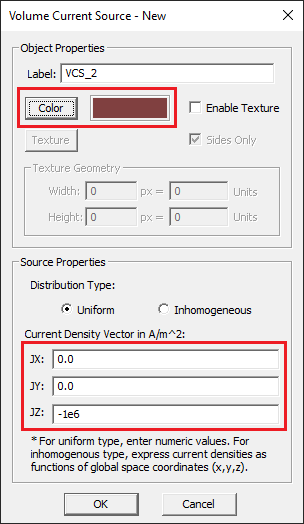The property dialog of the second volume current source group.

With the VCS_2 group active, draw a second upright cylinder according to the table below:

Part Object Type Material Type Dimensions Coordinates Rotation Angles
Cylinder_2 Cylinder VCS_2 radius = 2mm, height = 50mm (10mm, 0, -25mm) (0°, 0°, 0°)

Keep all the settings from the previous section. But change the location of the vertical "Sensor_2" plane as follows:

Field Sensor Direction Coordinates Plot Type Max. Size Cone Length Ratio Cone Radius Ratio
Sensor_2 X (5mm, 0, 0) Vector 2 0.25 0.25

Your physical structure should now look like this: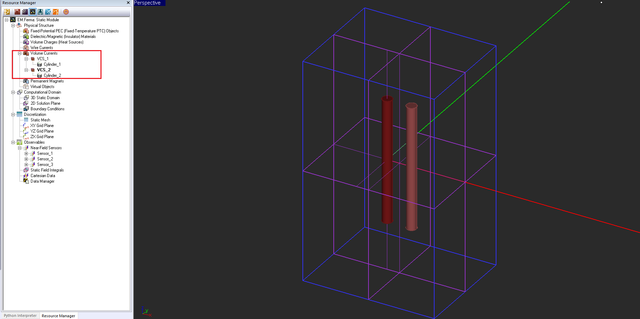The two cylindrical current objects along with the modified field sensor planes.

Run a magnetostatic simulation of your structure and visualize the field sensor plots.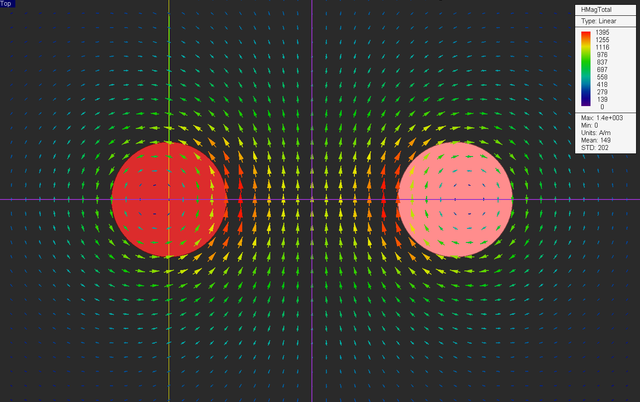The vector plot of the magnetic field distribution of the two parallel lines in the horizontal Sensor_1 plane.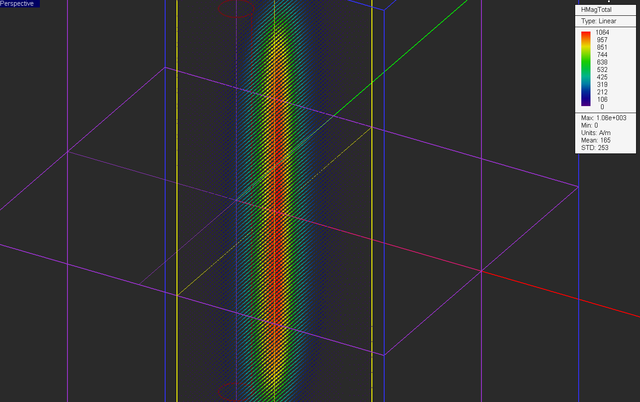The vector plot of the magnetic field distribution of the two parallel lines in the vertical Sensor_2 plane.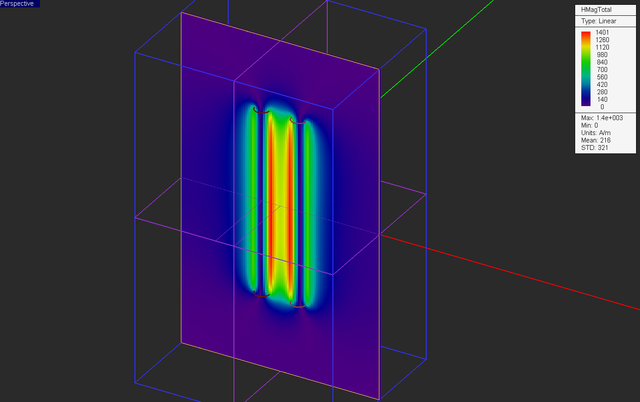The intensity plot of the total magnetic field distribution of the two parallel lines in the vertical Sensor_3 plane.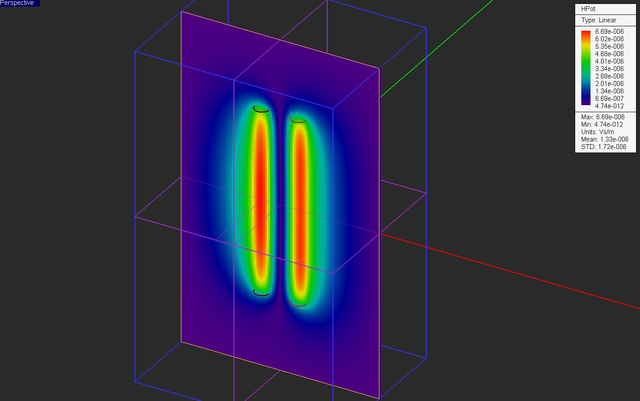The intensity plot of the magnitude of magnetic vector potential distribution of the two parallel lines in the vertical Sensor_3 plane.

Also plot the data file “Sensor_1_X_HTotal.DAT” and compare it to the previous graph of the single current case. Note how the magnetic fields of the two currents add up in the midway vertical plane and create a much higher field intensity on this plane.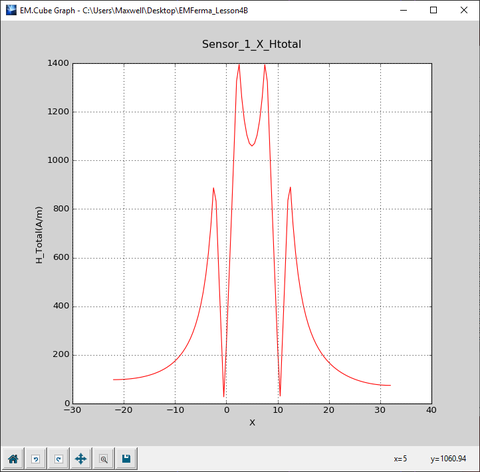The 2D Cartesian graph of variation of the magnetic of the two parallel lines along the X direction on the center horizontal plane.

## Adding a Magnetic Material Part to Your Structure

In this part of the tutorial lesson, you will add a fixture made of a magnetic material to hold your current cylinders. In EM.Ferma, dielectric and magnetic materials are lumped together as one category. For a magnetic material, you specify the relative permittivity μr. Define a new magnetic material group called "Magnetic_1" with the default light green and μr = 4.

With the new group "Magnetic_1" active in the navigation tree, draw two cylinders and a box object with the following specifications:

Part Object Type Material Type Dimensions Coordinates Rotation Angles
CYL_Left Cylinder Magnetic_1 radius = 3mm, height = 16mm (-4mm, 0, -8mm) (0°, 0°, 0°)
CYL_Right Cylinder Magnetic_1 radius = 3mm, height = 16mm (14mm, 0, -8mm) (0°, 0°, 180°)
Box_1 Box Magnetic_1 18mm × 6mm × 16mm (5mm, 0, -8mm) (0°, 0°, 0°)

Also, in the property dialogs of both cylinder objects "CYL_Left" and "CYL_Right", set the Start Angle to 90° and set the End Angle to 270°. This will turn these objects into half-cylinders. Note the second object has a Z rotation angle of 180°.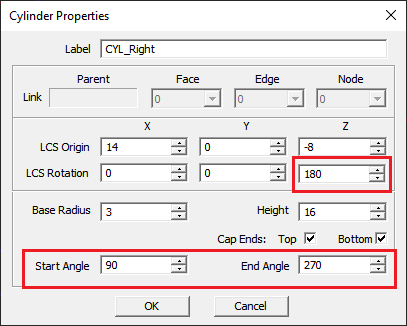The property dialog of the second half-cylinder "CYL_Right".

Keep all the other settings from the previous part intact including all the field sensor planes. Your physical structure should now look like this: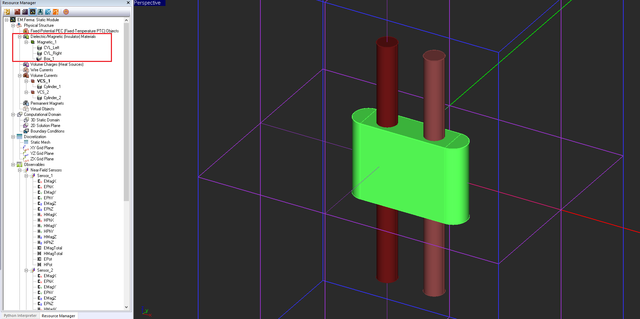The two cylindrical current objects along with the magnetic material fixture.

Using the same mesh settings from the previous parts, examine the fixed-cell mesh of your new structure.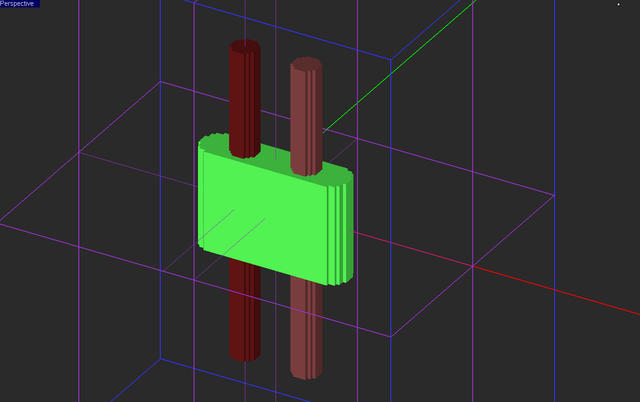The mesh of the modified structure with the added holding fixture.

Run a magnetostatic simulation of your structure and visualize the field sensor plots.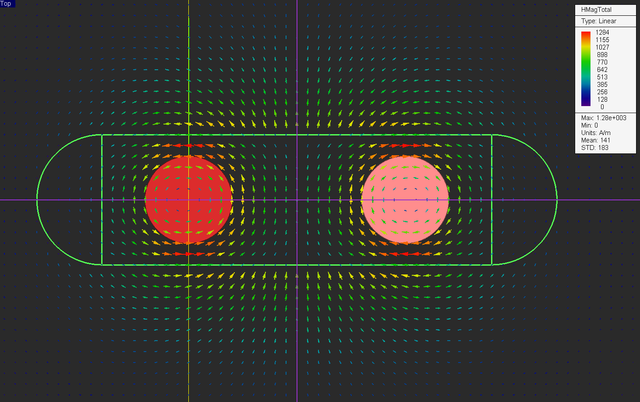The vector plot of the magnetic field distribution of the two parallel lines with the magnetic material fixture in the horizontal Sensor_1 plane.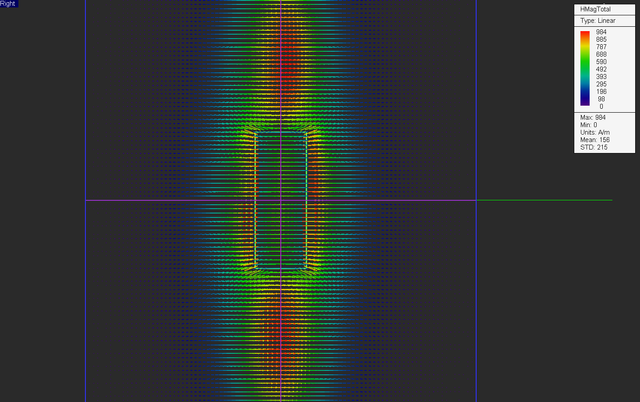The vector plot of the magnetic field distribution of the two parallel lines with the magnetic material fixture in the vertical Sensor_2 plane.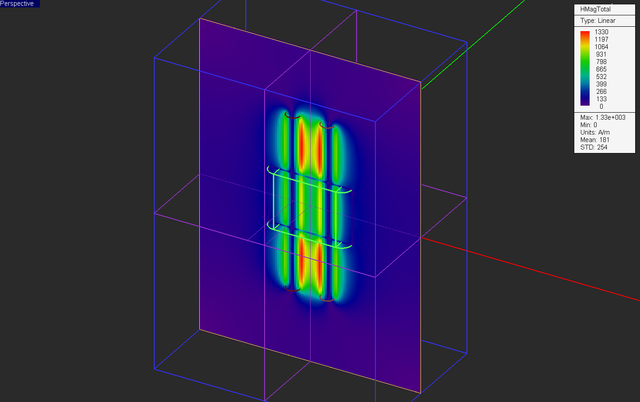The intensity plot of the total magnetic field distribution of the two parallel lines with the magnetic material fixture in the vertical Sensor_3 plane.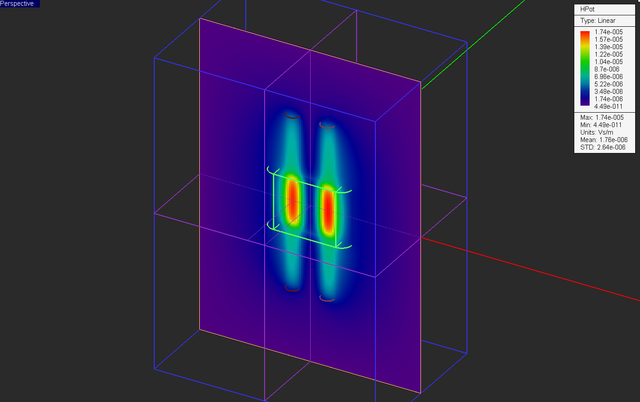The intensity plot of the magnitude of magnetic vector potential distribution of the two parallel lines with the magnetic material fixture in the vertical Sensor_3 plane.

Also plot the data file “Sensor_1_X_HTotal.DAT” and compare it to the previous graph of the case without the magnetic fixture. You can see that the magnetic field intensity at the center of the magnetic material (x = 5) has dropped down to 635A/m compared to the a much higher value of 1060A/m at the same spot in the previous case.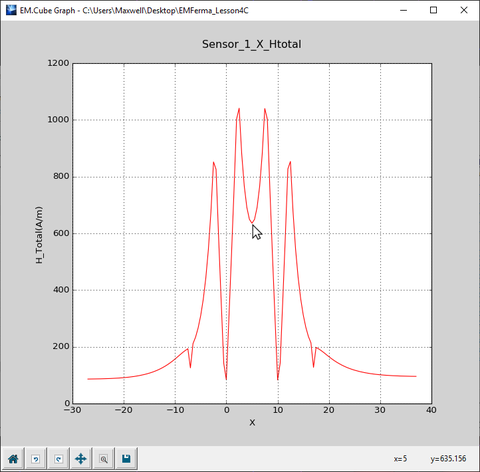The 2D Cartesian graph of variation of the magnetic of the two parallel lines with the magnetic material fixture along the X direction on the center horizontal plane.

## Testing a More Complicated Magnetic Material Part

In the structure of the previous part, a large portion of the two current-carrying cylinders overlap with the magnetic material fixture. The cylinders are sources (J) while the magnetic material is characterized by its permeability μ. Therefore, it was assumed that the current J flows in a material medium with the permeability μ = 4μ0 inside the two overlap regions. In the last part of this tutorial lesson, you are going to perforate two cylindrical holes in the magnetic holding fixture with the same radius as the current cylinders. As a result, the currents will flow in their entirety in an non-permeable medium with μ0.

To create the holes, activate the group "Magnetic_1" and draw two new cylinder objects as follows:

Part Object Type Material Type Dimensions Coordinates Rotation Angles
Hole_Left Cylinder Magnetic_1 radius = 2mm, height = 16mm (0, 0, -8mm) (0°, 0°, 0°)
Hole_Right Cylinder Magnetic_1 radius = 2mm, height = 16mm (10mm, 0, -8mm) (0°, 0°, 0°)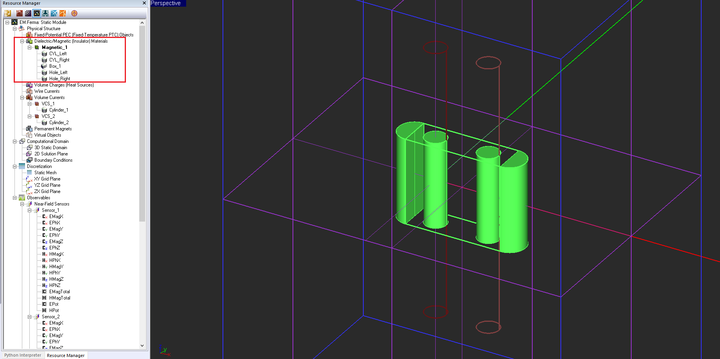The two new embedded cylinder objects shown with the enclosing object "Box_1" and the two current cylinders all in their freeze state.

The new cylinders are completely embedded inside "Box_1". Next, you are going to perform a Boolean subtraction operation. In this operation, you will subtract objects "Hole_Left" and "Hole_right" from the object "Box_1". Click the Subtract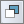button on the Action Toolbar.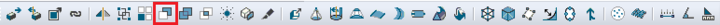Selecting the subtract tool on the "Tools" toolbar.

First click either on the surface of "Box_1" or on its name in the navigation tree to select the object to subtract form and press the keyboard's Enter key. Next select the names of "Hole_1" and "Hole_2" from the navigation tree. These are the objects to be subtracted. You can also click on the surface of these objects in the project workspace although it might be harder due to mouse-over conflicts. Since you can select multiple objects for subtraction, you have to press the keyboard's Enter key to signal the completion of the object selection.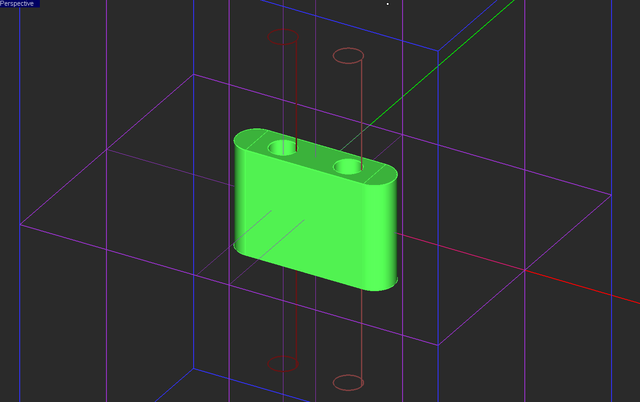The Boolean subtraction object shown with enclosing object "Box_1" in a freeze state.

The three objects "Box_1", "Hoe_Left" and "Hole_Right" are deleted from the navigation tree and replaced with a single Boolean object. You can right-click on the surface of the Boolean object or on its name in the navigation tree and open its property dialog from the contextual menu. Note that you can always access the constituent object and edit they individual properties.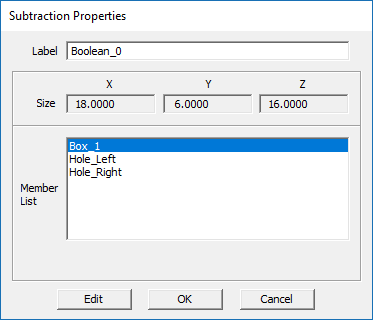The property dialog of the Boolean subtraction object.

With the Boolean operation completed, run a magnetostatic simulation of your modified structure and visualize the field sensor plots.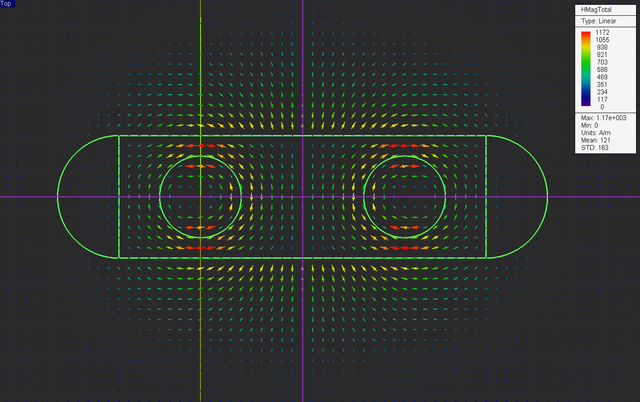The vector plot of the magnetic field distribution of the two parallel lines with the perforated magnetic material fixture in the horizontal Sensor_1 plane.The intensity plot of the total magnetic field distribution of the two parallel lines with the perforated magnetic material fixture in the vertical Sensor_3 plane.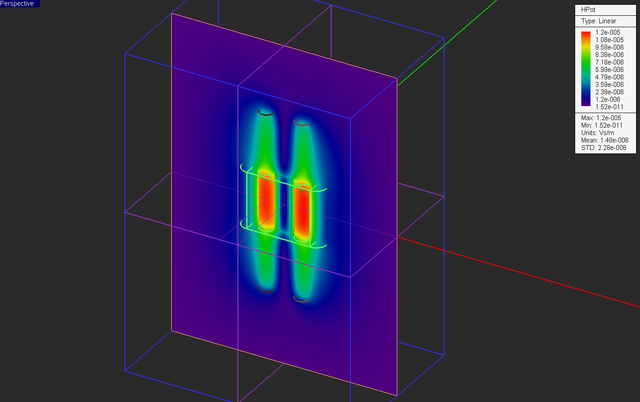The intensity plot of the magnitude of magnetic vector potential distribution of the two parallel lines with the perforated magnetic material fixture in the vertical Sensor_3 plane.

Also plot the data file “Sensor_1_X_HTotal.DAT” and compare it to the previous graph of the case the solid magnetic fixture. Note all the magnetic field discontinuities that occur at the air-material interfaces at x = -7mm, -2mm, 2mm, 8mm, 10mm and 17mm.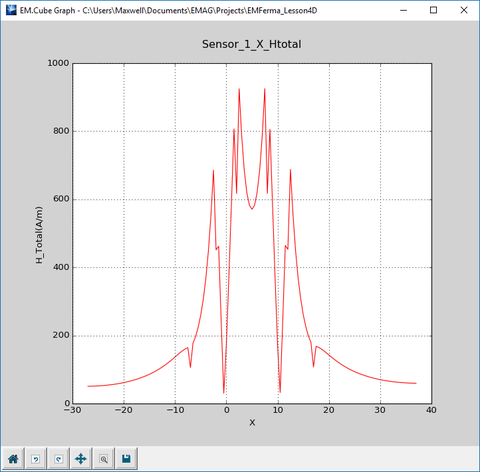The 2D Cartesian graph of variation of the magnetic of the two parallel lines with the perforated magnetic material fixture along the X direction on the center horizontal plane.